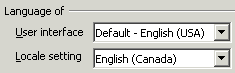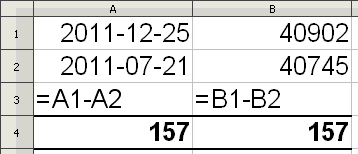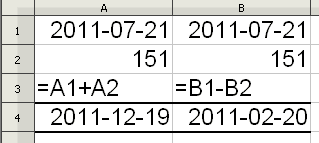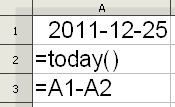Calc
Topics

# Calculating Dates

## Date Formats

### Language Settings

Before you can properly use dates in calculations, you need to know what whether Calc is using the USA or Canadian date Format. Many people aren't aware Americans and Canadians use different date formats:

• USA: Month/Day/Year - 4/5/11 would be read as April 5, 2011.
• Canada: Year/Month/Day - 4/5/11 would be converted to 2004-5-11 and read as May 11, 2004. Calc version 3 uses the strict Canadian Date format (Government) of Year/Month/Day and but there is also a general Canadian Date format of Day/Month/Year that earlier versions used. MS Excel uses the general Canadian date format.Therefore when using all numbers to represent the date instead of typing the month out as text, its important to know what is the default date format that Calc is using. The date format uses the Language Settings Panel to determine which format to use. This panel is part of the larger Options panel: TOOLS -> OPTIONS ->LANGUAGE SETTINGS -> LANUGUAGE -> LOCAL SETTINGS. In this example the date format used will be the Canadian date format because the local settings is set for English Canada. Calc version 3 uses the strict Canadian date format to represent the Canadian date. Since most Canadians don't write out a date using the strict format, its best when writing a date to type out the month, that way there is no confusing between user and Calc. If you are more comfortable with the USA date format of Month/Day/Year then change the Local Setting to English (USA).

### Changing a Date FormatRegardless of how you type a date, Calc always represents it in the default Short date format of all numbers (Canadian dates always displays as Year-Month-Day). However Calc supports many date formats, so if you would rather have a date with the month spelt out or in a another date format, then modify it through the Date category found in the Format Cells box (right click on the cell with the date and from the Context menu choose Format Cells, or choose Format Cells from the FORMAT menu). Don't be confused about the December 31, 1999 date shown in the Format window, it is just a representative sample of the different date formats.If you type a date in a cell and Calc doesn't convert it into its default Short date format of Year-Month-Day (for a Canadian date) then you probably typed the date wrong. Calc always aligns a proper date to the right in a cell as it does with numbers. If the typed date is not converted to the Short date format and/or is left aligned in the cell, then Calc is treating it as text, meaning that Calc doesn't recognize it as a date.

### Date Functions

Rather than typing a current date, a date function can be used. Two common date functions are:

• TODAY function: syntax: =TODAY() - always returns the current date ever time the sheet is opened
• NOW function: syntax: =NOW() - always returns the current date and updates the current time after every entry/calculation

Unlike most Calc functions there are no arguments in the brackets of these two functions, in other words the brackets () are empty. Occasionally when using these date functions instead of a date being returned you may get a long series of numbers, known as a serial number. If that happens Use the Format Cells menu and choose Date from the category list to convert the serial number back to a date format of your choice. Every date is really a serial number, that's how Calc can use dates in a calculation.

## Calculating the Days

### Writing the Date

Before you can use a date in a calculation it must be written in a format that Calc understands as a date. The Date category located in the Format Cells box (shown above) lists many variation that a date can be written. If using a short date (all numbers) it can written with "-", ".", or "/" between each field, for example July 21, 2011 can be written as: 11-7-21, 11.7.21, 11/7/21 for an English Canada date. Regardless how you write the Canadian date Calc will always display it as 2011-07-21.

If using only 2 fields for the date such as 7/21, Calc will interpret the first number as a month and the second number as the day and it will assume the current year. Entering 7/21 in a cell in the year 2011 will be convert to the default date of 2011-07-21. If using only 2 digits to represent a year, Calc will interpret "30-99" as the years 1930-1999. The years "00-29" will be interpreted as 2000-2029.

### The Serial Number behind the Date

No special formula is required to calculate the number of days between two dates or to calculate a future date by adding a number of days to the current date. Same formula rules of adding and subtracting apply to dates also. The reason there is no difference in the formula for calculating dates is because every date is really a serial number. If you use the Format Cells box to convert a Date format to the Number format, you will see the date's serial number.

July 21, 2011 has a serial number of 40745. Where does that number come from? It's quite simple, in order to calculate dates Calc assigns a serial number to every date. But it has to start somewhere and it chooses December 30, 1899 as serial number 0. Therefore, December 31, 1899 is serial number 1 and so forth to the present date and beyond. The following illustration displays simple date calculation between dates formats and their serial numbers counterparts. The formula calculates the number of days to Christmas between July 21, 2011 and December 25, 2011.Column A display the two dates and the simple subtraction formula that results in 157.
Column B displays the same data but has the dates converted into their respective serial numbers. Remember that the serial number 1 start at December 31, 1899, therefore, counting the number of days forward from December 31, 1899 to December 25, 2011 results in 40,902 days and 40,745 days for July 21, 2011.

As this illustration demonstrates there is no mystery in how dates are calculated. Every date has serial number associated with it with the arbitrary date of December 31, 1899 being serial number 1. Counting backwards from December 30, 1899 (serial number 0) results in a negative number. Therefore December 29, 1899 would be serial number -1. So calculating dates really is just calculating numbers, the difference is that you don't normally see the serial numbers behind the date but every date is really a serial number.

You will also notice in the above illustration that the formula references the cells that contain the dates. You do not type a date directly onto the formula! The formula above is correctly written as "=A1-A2" with A1 referencing the first date and A2 referencing the second date. However if the dates were typed directly into the formula as "=2011-12-25-2011-07-21" the result would be -38. This is because Calc will interpret the numbers in the formula as numbers, not as a date, and the hyphen between each set of numbers will be read as subtraction operator not as a separator. Calc will then proceed to subtract the numbers starting from the left. About the only time a date can be typed directly into a formula is if the date is converted into its serial number or is typed as a date function.

### Writing the formula

Keeping in mind that behind every date is a serial number, it is not likely that you will ever be adding, multiplying or dividing two dates directly. In the above illustration if you were to add the two dates "=A1+A2" the result would yield a serial number of 81647 (40902+40745), and when converted back to a date would results in a bizarre future date of 2123-07-16.

As a result, when calculating between two dates you will invariable be subtracting the dates. However, as in all subtraction formulas, the order of subtraction is important. It will always be the bigger date minus the smaller date. In the illustration above the bigger date is 2011-12-25 (December 25, 2011) because its serial number is larger than the 2011-07-21 (July 21, 2011) serial number. If the formula is reversed "=A2-A1", the result will yield a negative number: -157.

To find a date in the future, add the number of days to the date. To find a date in the past, subtract the number of days from the date. For example to find out what the date will be 151 days from 2011-07-21 (July 21, 2011) , add 151 to that date. To find out what the date was 151 from 2011-07-21 (July 21, 2011), subtract 151 from that date.In Column A 151 days are added to the date of 2011-07-21 (July 21, 2011) resulting in the future date of 2011-12-19 (December 19, 2011).

In Column B 151 days are subtracted from the date of 2011-07-21 (July 21, 2011) resulting in the past date of 2011-02-20 (February 20, 2011).

Date functions can be typed directly into formulas if needed. The formula "=TODAY()+151" would calculate the date 151 days from the current date. Since the TODAY() function always displays the current date, this formula would constantly change, always displaying the date 151 days from the current date every time the spreadsheet was opened.Likewise if you wanted to do a countdown, for example counting down the number of days to Christmas, the formula would be the Christmas date minus the TODAY() function (as shown). Every time the spreadsheet was opened the formula result would be a smaller number because the TODAY() function always inserts the current date and the current date would be getting closer and closer to the Christmas date.

If the spreadsheet were opened after the Christmas date the formula result would be a negative number. Remember when subtracting dates, its always the bigger serial number date minus the smaller serial number date. In this example the bigger serial number date is the Christmas date and the smaller serial number date is the TODAY() function. However, after Christmas passes the reverse becomes true.

## Summary

Its a simple process to calculate using dates. Keep these points in mind:

• Determine whether Calc is using the USA date format or one of the two Canadian date formats (Calc version 3 uses the strict Canadian date format).
• Ensure that the date is written in a proper date format.
• Remember that every date is a serial number and the formula really just calculates the serial number.
• When subtracting between two dates its always the latest/future date (bigger serial number) minus the earlier/past date (lower serial number).
• Its best to use cell references when calculate dates rather than typing a date directly into a formula.